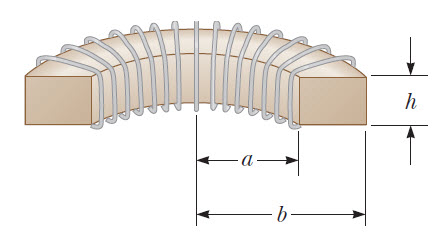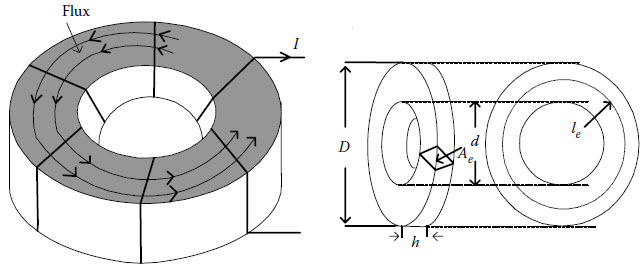# Toroid Inductor Online Calculator

Below is online calculator for calculating inductance or number of turns required to make iron core and ferrite toroid inductor. There are two calculators- the first one uses the inductance index(inductance factor) and the other uses the geometry of the toroid.

### A. Using Inductance Factor $$A_L$$

Calculate N:

Result:

Calculate L:

Result:#### Iron Powered Toroid Inductor

The formula for Iron Powered Toroid Inductor used above is given below.

Inductance(L):  $$L_ = \frac{A_L N^2}{10,000} [\mu H]$$

Number of Turns(N):  $$N = 100 \sqrt{\frac{L_{\mu H}}{A_L}}$$

where,
- L is inductance in uH
- $$A_L$$ is inductance factor(inductance index) in $$\mu H$$ per 100 turn squared

#### Ferrite Toroid Inductor

The formula for Ferrite Toroid Inductor used above is given below.

Inductance(L):  $$L = \frac{A_L N^2}{1,000,000} [mH]$$

Number of Turns(N):  $$N = 1000 \sqrt{\frac{L_{mH}}{A_L}}$$

where,
- L is inductance in mH
- $$A_L$$ is inductance factor(inductance index) in mH per 1,000 turn squared

### B. Using Toroid Geometry

Geometry based Toroid Inductor Online Calculator
Calculate N:

cm
cm
cm

Result:

cm2
cm

Calculate L:
cm
cm
cm

Result:

cm2
cmFormula Used:

Inductance:  $$L = \frac{4 \pi N^2 \mu_i n A_e}{l_e}[nH]$$

Effective Length:  $$l_e = \frac{ \pi (D-d)}{ln(\frac{D}{d})} [cm^2]$$

Effective Area:  $$A_e = 0.5 n(D-d) h[cm]$$

where,
- L is inductance in nH
- D is the outer diameter in cm
- d is the inner diameter in cm
- h is the height in cm
- $$A_e$$ is the effective area in $$cm^2$$
- $$l_e$$ is the effective length in cm
- $$\mu_i$$ is the initial permeability
- N is the number of turns
- n is the number of stacked cores